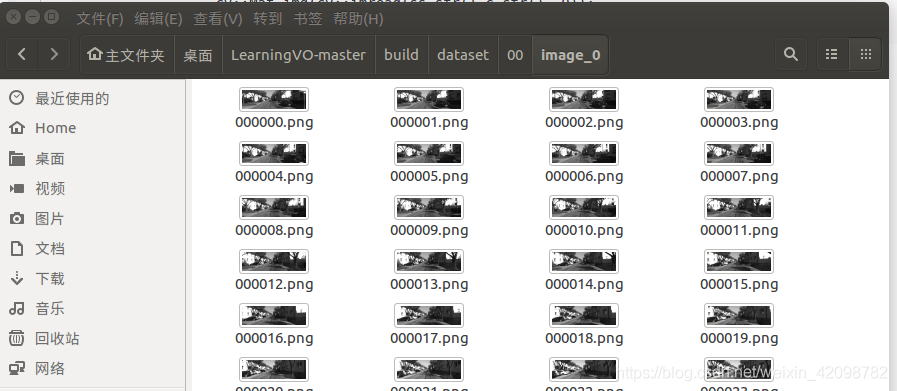program code is as follows:

``````		// 导入图像
std::stringstream ss;
ss <<  "/home/wang/桌面/LearningVO-master/build/dataset/00/image_0/"
<< std::setw(6) << std::setfill('0') << img_id << ".png";

assert(!img.empty());``````

picture path is as follows:but the program will assert failed.

my solution:

OpenCV absolute path seems to be a problem, change the absolute path to relative path:

``````		// 导入图像
std::stringstream ss;
ss <<  "./dataset/00/image_0/"
<< std::setw(6) << std::setfill('0') << img_id << ".png";